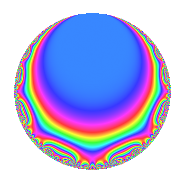# Properties

 Label 4012.2.a.fLevel 4012 Weight 2 Character orbit 4012.a Self dual yes Analytic conductor 32.036 Analytic rank 1 Dimension 3 CM no Inner twists 1

# Related objects

## Newspace parameters

 Level: $$N$$ = $$4012 = 2^{2} \cdot 17 \cdot 59$$ Weight: $$k$$ = $$2$$ Character orbit: $$[\chi]$$ = 4012.a (trivial)

## Newform invariants

 Self dual: yes Analytic conductor: $$32.0359812909$$ Analytic rank: $$1$$ Dimension: $$3$$ Coefficient field: 3.3.321.1 Coefficient ring: $$\Z[a_1, \ldots, a_{23}]$$ Coefficient ring index: $$2^{2}$$ Twist minimal: yes Fricke sign: $$1$$ Sato-Tate group: $\mathrm{SU}(2)$

## $q$-expansion

Coefficients of the $$q$$-expansion are expressed in terms of a basis $$1,\beta_1,\beta_2$$ for the coefficient ring described below. We also show the integral $$q$$-expansion of the trace form.

 $$f(q)$$ $$=$$ $$q - q^{3} -\beta_{1} q^{5} + q^{7} -2 q^{9} +O(q^{10})$$ $$q - q^{3} -\beta_{1} q^{5} + q^{7} -2 q^{9} -2 q^{11} + ( 1 + \beta_{1} ) q^{13} + \beta_{1} q^{15} + q^{17} + ( -2 + \beta_{1} ) q^{19} - q^{21} + ( -\beta_{1} - \beta_{2} ) q^{23} + ( 6 + 2 \beta_{2} ) q^{25} + 5 q^{27} + ( -2 - \beta_{1} ) q^{29} + ( 2 + \beta_{1} - \beta_{2} ) q^{31} + 2 q^{33} -\beta_{1} q^{35} + ( 3 + 2 \beta_{1} - \beta_{2} ) q^{37} + ( -1 - \beta_{1} ) q^{39} + ( -2 + \beta_{1} ) q^{41} + ( -2 + \beta_{1} - \beta_{2} ) q^{43} + 2 \beta_{1} q^{45} + ( -4 + \beta_{1} + \beta_{2} ) q^{47} -6 q^{49} - q^{51} + ( 5 + 2 \beta_{2} ) q^{53} + 2 \beta_{1} q^{55} + ( 2 - \beta_{1} ) q^{57} - q^{59} + ( -3 - \beta_{1} ) q^{61} -2 q^{63} + ( -11 - \beta_{1} - 2 \beta_{2} ) q^{65} + ( -2 + 2 \beta_{1} + 2 \beta_{2} ) q^{67} + ( \beta_{1} + \beta_{2} ) q^{69} + ( -2 + 2 \beta_{2} ) q^{71} + 2 q^{73} + ( -6 - 2 \beta_{2} ) q^{75} -2 q^{77} -5 q^{79} + q^{81} + ( -1 + 2 \beta_{1} - \beta_{2} ) q^{83} -\beta_{1} q^{85} + ( 2 + \beta_{1} ) q^{87} + ( 4 + \beta_{1} - \beta_{2} ) q^{89} + ( 1 + \beta_{1} ) q^{91} + ( -2 - \beta_{1} + \beta_{2} ) q^{93} + ( -11 + 2 \beta_{1} - 2 \beta_{2} ) q^{95} + ( -3 + \beta_{1} - 2 \beta_{2} ) q^{97} + 4 q^{99} +O(q^{100})$$ $$\operatorname{Tr}(f)(q)$$ $$=$$ $$3q - 3q^{3} + q^{5} + 3q^{7} - 6q^{9} + O(q^{10})$$ $$3q - 3q^{3} + q^{5} + 3q^{7} - 6q^{9} - 6q^{11} + 2q^{13} - q^{15} + 3q^{17} - 7q^{19} - 3q^{21} + 20q^{25} + 15q^{27} - 5q^{29} + 4q^{31} + 6q^{33} + q^{35} + 6q^{37} - 2q^{39} - 7q^{41} - 8q^{43} - 2q^{45} - 12q^{47} - 18q^{49} - 3q^{51} + 17q^{53} - 2q^{55} + 7q^{57} - 3q^{59} - 8q^{61} - 6q^{63} - 34q^{65} - 6q^{67} - 4q^{71} + 6q^{73} - 20q^{75} - 6q^{77} - 15q^{79} + 3q^{81} - 6q^{83} + q^{85} + 5q^{87} + 10q^{89} + 2q^{91} - 4q^{93} - 37q^{95} - 12q^{97} + 12q^{99} + O(q^{100})$$

Basis of coefficient ring in terms of a root $$\nu$$ of $$x^{3} - x^{2} - 4 x + 1$$:

 $$\beta_{0}$$ $$=$$ $$1$$ $$\beta_{1}$$ $$=$$ $$2 \nu - 1$$ $$\beta_{2}$$ $$=$$ $$2 \nu^{2} - 2 \nu - 5$$
 $$1$$ $$=$$ $$\beta_0$$ $$\nu$$ $$=$$ $$($$$$\beta_{1} + 1$$$$)/2$$ $$\nu^{2}$$ $$=$$ $$($$$$\beta_{2} + \beta_{1} + 6$$$$)/2$$

## Embeddings

For each embedding $$\iota_m$$ of the coefficient field, the values $$\iota_m(a_n)$$ are shown below.

For more information on an embedded modular form you can click on its label.

Label $$\iota_m(\nu)$$ $$a_{2}$$ $$a_{3}$$ $$a_{4}$$ $$a_{5}$$ $$a_{6}$$ $$a_{7}$$ $$a_{8}$$ $$a_{9}$$ $$a_{10}$$
1.1
 2.46050 0.239123 −1.69963
0 −1.00000 0 −3.92101 0 1.00000 0 −2.00000 0
1.2 0 −1.00000 0 0.521753 0 1.00000 0 −2.00000 0
1.3 0 −1.00000 0 4.39926 0 1.00000 0 −2.00000 0
 $$n$$: e.g. 2-40 or 990-1000 Significant digits: Format: Complex embeddings Normalized embeddings Satake parameters Satake angles

## Inner twists

This newform does not admit any (nontrivial) inner twists.

## Twists

By twisting character orbit
Char Parity Ord Mult Type Twist Min Dim
1.a even 1 1 trivial 4012.2.a.f 3

By twisted newform orbit
Twist Min Dim Char Parity Ord Mult Type
4012.2.a.f 3 1.a even 1 1 trivial

## Atkin-Lehner signs

$$p$$ Sign
$$2$$ $$-1$$
$$17$$ $$-1$$
$$59$$ $$1$$

## Hecke kernels

This newform subspace can be constructed as the intersection of the kernels of the following linear operators acting on $$S_{2}^{\mathrm{new}}(\Gamma_0(4012))$$:

 $$T_{3} + 1$$ $$T_{5}^{3} - T_{5}^{2} - 17 T_{5} + 9$$